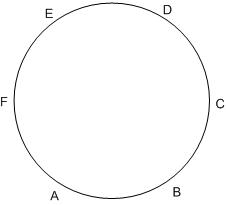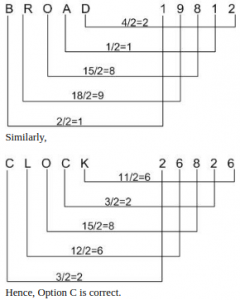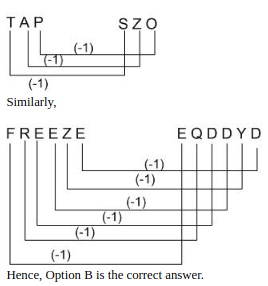0
3722

# Logical Reasoning Questions For SSC CGL PDF:

Download Logical Reasoning Questions For SSC CGL PDF with solutions. Important practice problems on profit, loss and discount based on asked questions in CGL Previous papers.

Question 1: The heights of three towers are in the ratio 5 : 6 : 7. If a spider takes 15 minutes to climb the smallest tower, how much time will it take to climb the highest one?

a) 15 minutes
b) 18 minutes
c) 21 minutes
d) 54 minutes

Question 2: Six friends A, B, C, D, E and F are sitting in a circle. C is to the left of D, F is between A and E, and E is between F and D. Who is to the left of F?

a) A
b) C
c) D
d) E

Question 3: Given below are the jumbled letters of a word and their corresponding numbers. Select the combination of numbers that makes a meaningful word.

C N A S P H I
1 2 3 4 5 6 7

a) 5742613
b) 2375416
c) 4572316
d) 7234516

Question 4: If BROAD means 19812, CLOCK means

a) 68262
b) 68622
c) 26826
d) 37836

Question 5: TAP is SZO, then FREEZE is

a) EQDFYG
b) EQDDYD
c) ESDFYF
d) GQFDYF

We know that distance=Speed $\times$ Time.
If speed is constant than,
$\frac{Distance}{Time}$=constant.
Let height of smallest tower be ‘$5x$’.
$\therefore$ height of largest tower will be ‘$7x$’.
Let time taken by spider to climb largest time be ‘t’ minutes.
$\frac{5x}{15}=\frac{7x}{t}$
$t=21$
So, time taken by spider to climb largest tower will be 21 minutes.
Hence, Option C is correct.Clearly, E is to the left of F.
Hence, Option D is correct.

Considering Options,
Option A 5742613= ‘PISNHCA’.
Option B 2375416= ‘NAIPSCH’.
Option C 4572316= ‘SPINACH’.
Option D 7234516= ‘INASPCH’.
Clearly, Only Option C i.e. SPINACH makes sense.
All other options are incorrect.
Hence, Option C is correct